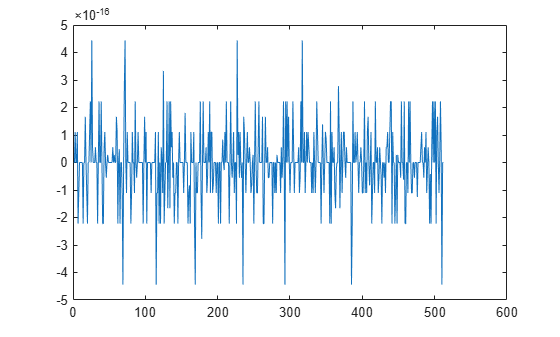# wdf2allpass

Wave Digital Filter to allpass coefficient transformation

## Syntax

``a = wdf2allpass(w)``
``A = wdf2allpass(W)``

## Description

````a = wdf2allpass(w)` accepts a vector of transformed real allpass coefficients, `w`, and returns the conventional allpass polynomial version `a`. `w` is used by allpass filter objects such as `dsp.AllpassFilter`, and `dsp.CoupledAllpassFilter`, with `Structure` set to `'Wave Digital Filter'`. ```
````A = wdf2allpass(W)` accepts the cell array of transformed allpass coefficient vectors `W`. Each cell of `W` contains the transformed real coefficients of a section of a cascade allpass filter. The output `A` is also a cell array, and each cell of `A` contains the conventional polynomial version of the corresponding cell of `W`. `W` is used by allpass filter objects such as `dsp.AllpassFilter` and `dsp.CoupledAllpassFilter`, with `Structure` set to `'Wave Digital Filter'`. Every cell of `W` must contain a real vector of length 1,2, or 4. When the length is 4, the second and fourth components must both be zero. `W` can be a row or column vector of cells while `A` is always returned as column.```

## Examples

collapse all

Note: If you are using R2016a or earlier, replace each call to the object with the equivalent `step` syntax. For example, `obj(x)` becomes `step(obj,x)`.

Create a second order allpass filter with wave digital filter coefficients w = [0.5 0]. Convert these coefficients into polynomial form using `wdf2allpass`. Assign the polynomial coefficients to an allpass filter using the 'Minimum multiplier' structure. Pass a random input to both these filters and compare the outputs.

```w = [0.5 0]; allpasswdf = dsp.AllpassFilter('Structure', 'Wave Digital Filter',... 'WDFCoefficients', w); a = wdf2allpass(w); allpass = dsp.AllpassFilter('AllpassCoefficients', a); in = randn(512, 1); outputallpasswdf = allpasswdf(in); outputallpass = allpass(in); plot(outputallpasswdf-outputallpass)```The difference between the two outputs is very small.

## Input Arguments

collapse all

Numeric vector of transformed Wave Digital Filter allpass coefficients, specified as a real number. `w` can have only length equal to 1,2, and 4. When the length is 4, the second and fourth components must both be zero. `w` can be a row or a column vector.

Example: `[0.3,-0.2]`

Data Types: `double` | `single`

Cascade of allpass filter coefficients in transformed Wave Digital Filter form, specified as a cell vector. Every cell of `W` must contain a real vector of length 1, 2, or 4. When the length is 4, the second and fourth components must both be zero. `W` can be a row or a column vector of cells.

Example: `{[0.3,-0.2];0.5}`

## Output Arguments

collapse all

Numeric vector of polynomial allpass coefficients, determined as a numeric row vector.

Data Types: `double` | `single`

Cascade of allpass filter coefficient, determined as a column of cells, each containing a vector of length 1, 2, or 4.

Example: `{0.3 5.0 0.2}`

Data Types: `double` | `single`

## Algorithms

`wdf2allpass` provides the inverse operation of `allpass2wdf`, by transforming the transformed cascade of allpass coefficients `W` into their conventional polynomial representation `A`. Please refer to the reference page for `allpass2wdf` for more details about the two representations.

`W` defines a multisection allpass filter, and `wdf2allpass` applies separately to each section, with the same transformation used in the single-section case. In this case, the numeric coefficients vector `w` can have order 1, 2, or 4.

The relations between the vector of section coefficients a and w respectively depend on the order, as follows:

 M. Lutovac, D. Tosic, B. Evans, Filter Design for Signal Processing using MATLAB and Mathematica. Prentice Hall, 2001.